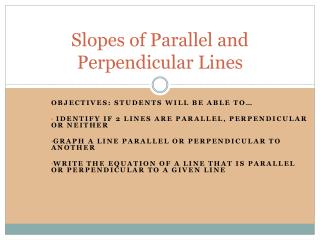Download PresentationSlopes of Parallel and Perpendicular Lines

# Slopes of Parallel and Perpendicular Lines

Download Presentation## Slopes of Parallel and Perpendicular Lines

- - - - - - - - - - - - - - - - - - - - - - - - - - - E N D - - - - - - - - - - - - - - - - - - - - - - - - - - -
##### Presentation Transcript

1. Slopes of Parallel and Perpendicular Lines Objectives: Students will be able to… identify if 2 lines are parallel, perpendicular or neither Graph a line parallel or perpendicular to another Write the equation of a line that is parallel or perpendicular to a given line

2. What do you know about parallel lines??

3. Slopes of Parallel Lines Slopes of parallel lines are equal Any 2 vertical lines are parallel; Any 2 horizontal lines are parallel Test whether 2 non-vertical or non-horizontal lines are || by comparing slopes

4. Determining if 2 lines are parallel on the coordinate plane EXAMPLE: l1 contains points A(1,5) and B(3,1). l2contains points C(3,3) and D(1, -4) Are the 2 lines parallel? Explain. Step 1: find the slope of both lines using slope formula: Step 2: Compare the slopes…are they the same? If yes, then the lines are parallel, if no, then they are not.

5. Are the two lines parallel? Line 1 contains P(0,3) and Q(-2, 5) Line 2 contains R(0,-7) and S(3,-10)

6. Are the lines 4y-12x=20 and y=3x-1 parallel? Explain. Compare Slopes by re-writing in slope-intercept form: y=mx+b • Isolate y • Remember, “m” is the slope, not “mx”

7. Are the following lines parallel: 6x – 12y=8 and 2y = 4x -7

8. Graph a line parallel to the given line:

9. The following lines are perpendicular. Calculate the slopes. What do you notice?

10. Slope and Perpendicular Lines Perpendicular Lines have slopes that are opposite, reciprocals of each other Ex) Slope:Perpendicular slope: 2 -1/2 1/3 -3 The product of perpendicular slopes is -1 Any vertical and any horizontal line are perp.

11. Are the 2 lines perpendicular? To answer this question, do the following: Find their slopes If their slopes are opposite, reciprocal, then they are perpendicular. Example: line 1: (-2,3) and (6, -3) line 2: (-3,-2) and (0,2)

12. Are the 2 lines perpendicular: Rewrite in slope int. form and compare slopes. 1. 2x-y=5 y= -1/2x +7 y=5 x=-2 3. -3x+y=5 3x –y =4

13. Graph a line perpendicular to the given line.

14. Writing Equations of Parallel Lines Write an equation of a line parallel to y=-2x +3 that contains the point (1, -2). Step 1: Identify slope in given line Step 2: Use the given slope ONLY and the given point ONLY to write the equation (y = mx +b) • Substitute m, x and y into y = mx + b • Solve for b: • Write equation using the m and the new b only:

15. EXAMPLE Write the equation of a line parallel to y = ½ x +4 that passes through the point (-4, 3).

16. Writing equations for perpendicular lines. Identify slope of given line. Find the slope of the line perpendicular to the given line (opposite, reciprocal) Use the slope from step 2 ONLY and given point ONLY to write new equation.

17. Write the equation for the line perpendicular to y=-3x-5 that contains the point (-3, 7). 1. Identify slope of given line: 2. Write the perpendicular slope (this is what you use for the rest of the problem): 3. Use the slope from step 2 and the x and y given to write equation:

18. Example: Write the equation of the line perpendicular to the line y=4x +1 that passes through the point (4, -5).

19. Another Form of a Line (EASY EASY!!) POINT SLOPE FORM: y - y1 = m(x - x1) m = slope (x1, y1) is a point on the line Use if you know the slope of a line and one point.

20. Let’s write these equations in point-slope form… 1. Write the equation of a line parallel to y = ½ x +4 that passes through the point (-4, 3). Identify slope of given line: Use point give and slope to write line: 2. Write the equation of the line perpendicular to the line y=4x +1 that passes through the point (4, -5). Identify slope of given line: Write perpendicular slope: Use perp. Slope and point to write equation: